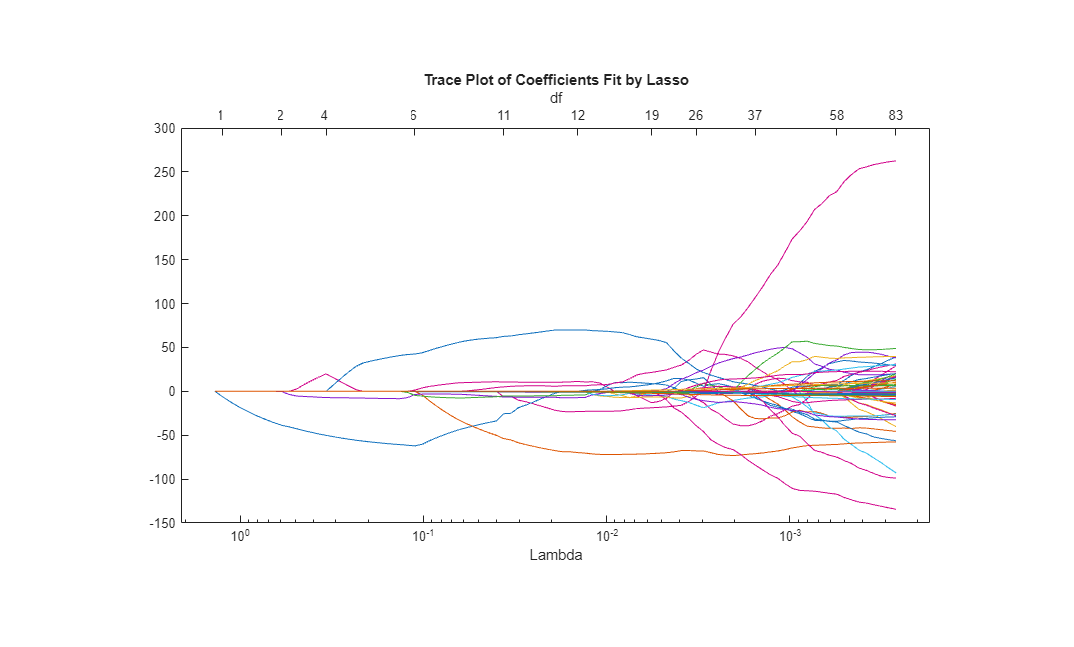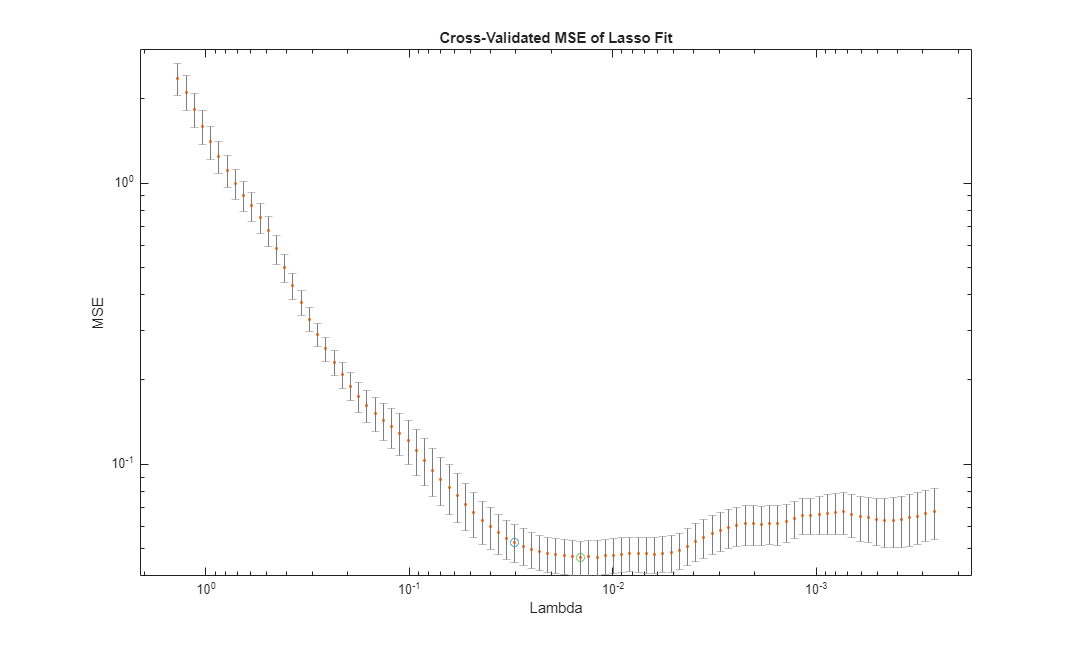Documentation

## Wide Data via Lasso and Parallel Computing

This example shows how to use `lasso` along with cross validation to identify important predictors.

Load the sample data and display the description.

```load spectra Description ```
```Description = 11×72 char array '== Spectral and octane data of gasoline == ' ' ' 'NIR spectra and octane numbers of 60 gasoline samples ' ' ' 'NIR: NIR spectra, measured in 2 nm intervals from 900 nm to 1700 nm ' 'octane: octane numbers ' 'spectra: a dataset array containing variables for NIR and octane ' ' ' 'Reference: ' 'Kalivas, John H., "Two Data Sets of Near Infrared Spectra," Chemometrics' 'and Intelligent Laboratory Systems, v.37 (1997) pp.255-259 ' ```

Lasso and elastic net are especially well suited for wide data, that is, data with more predictors than observations with lasso and elastic net. There are redundant predictors in this type of data. You can use `lasso` along with cross validation to identify important predictors.

Compute the default `lasso` fit.

```[b fitinfo] = lasso(NIR,octane); ```

Plot the number of predictors in the fitted lasso regularization as a function of `Lambda` , using a logarithmic x -axis.

```lassoPlot(b,fitinfo,'PlotType','Lambda','XScale','log'); ```It is difficult to tell which value of `Lambda` is appropriate. To determine a good value, try fitting with cross validation.

```tic [b fitinfo] = lasso(NIR,octane,'CV',10); toc ```
```Elapsed time is 7.353767 seconds. ```

Plot the result.

```lassoPlot(b,fitinfo,'PlotType','Lambda','XScale','log'); ```Display the suggested value of `Lambda` .

```fitinfo.Lambda1SE ```
```ans = 0.0302 ```

Display the `Lambda` with minimal MSE.

```fitinfo.LambdaMinMSE ```
```ans = 0.0144 ```

Examine the quality of the fit for the suggested value of `Lambda` .

```lambdaindex = fitinfo.Index1SE; mse = fitinfo.MSE(lambdaindex) df = fitinfo.DF(lambdaindex) ```
```mse = 0.0528 df = 11 ```

The fit uses just 11 of the 401 predictors and achieves a small cross-validated MSE.

Examine the plot of cross-validated MSE.

```lassoPlot(b,fitinfo,'PlotType','CV'); % Use a log scale for MSE to see small MSE values better set(gca,'YScale','log'); ```As `Lambda` increases (toward the left), MSE increases rapidly. The coefficients are reduced too much and they do not adequately fit the responses. As `Lambda` decreases, the models are larger (have more nonzero coefficients). The increasing MSE suggests that the models are overfitted.

The default set of `Lambda` values does not include values small enough to include all predictors. In this case, there does not appear to be a reason to look at smaller values. However, if you want smaller values than the default, use the `LambdaRatio` parameter, or supply a sequence of `Lambda` values using the `Lambda` parameter. For details, see the `lasso` reference page.

Cross validation can be slow. If you have a Parallel Computing Toolbox license, speed the computation of cross-validated lasso estimate using parallel computing. Start a parallel pool.

```mypool = parpool() ```
```Starting parallel pool (parpool) using the 'local' profile ... connected to 6 workers. mypool = Pool with properties: Connected: true NumWorkers: 6 Cluster: local AttachedFiles: {} AutoAddClientPath: true IdleTimeout: 30 minutes (30 minutes remaining) SpmdEnabled: true ```

Set the parallel computing option and compute the lasso estimate.

```opts = statset('UseParallel',true); tic; [b fitinfo] = lasso(NIR,octane,'CV',10,'Options',opts); toc ```
```Elapsed time is 3.799009 seconds. ```

Computing in parallel using two workers is faster on this problem.

Stop parallel pool.

```delete(mypool) ```
```Parallel pool using the 'local' profile is shutting down. ```